# Electric Field 1 Electric Field The electric field

• Slides: 61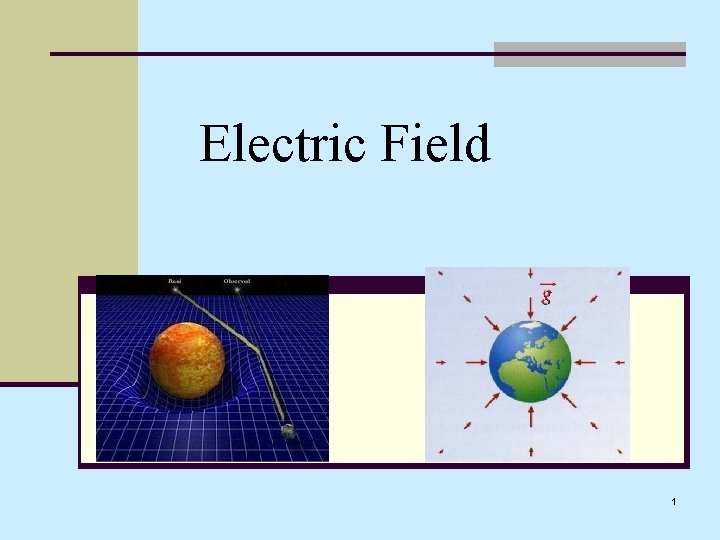Electric Field 1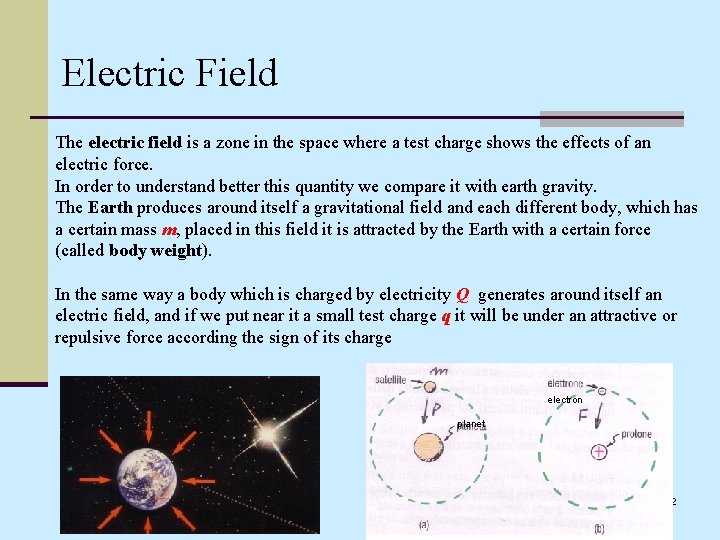Electric Field The electric field is a zone in the space where a test charge shows the effects of an electric force. In order to understand better this quantity we compare it with earth gravity. The Earth produces around itself a gravitational field and each different body, which has a certain mass m, placed in this field it is attracted by the Earth with a certain force (called body weight). In the same way a body which is charged by electricity Q generates around itself an electric field, and if we put near it a small test charge q it will be under an attractive or repulsive force according the sign of its charge electron planet 2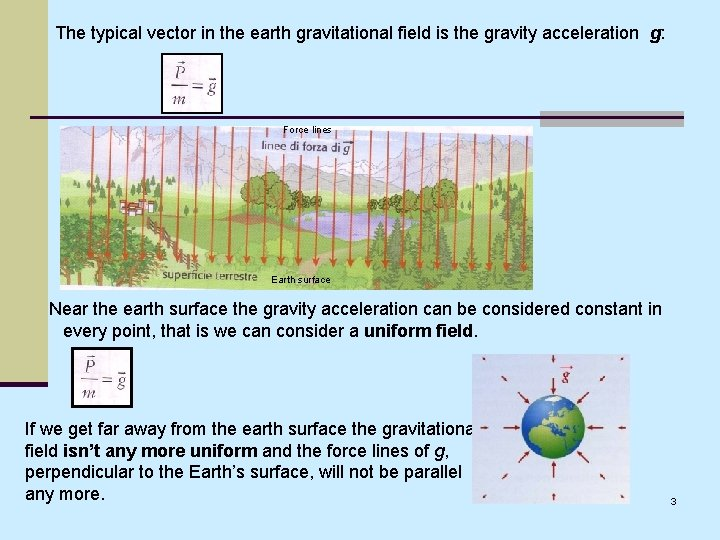The typical vector in the earth gravitational field is the gravity acceleration g: Force lines Earth surface Near the earth surface the gravity acceleration can be considered constant in every point, that is we can consider a uniform field. If we get far away from the earth surface the gravitational field isn’t any more uniform and the force lines of g, perpendicular to the Earth’s surface, will not be parallel any more. 3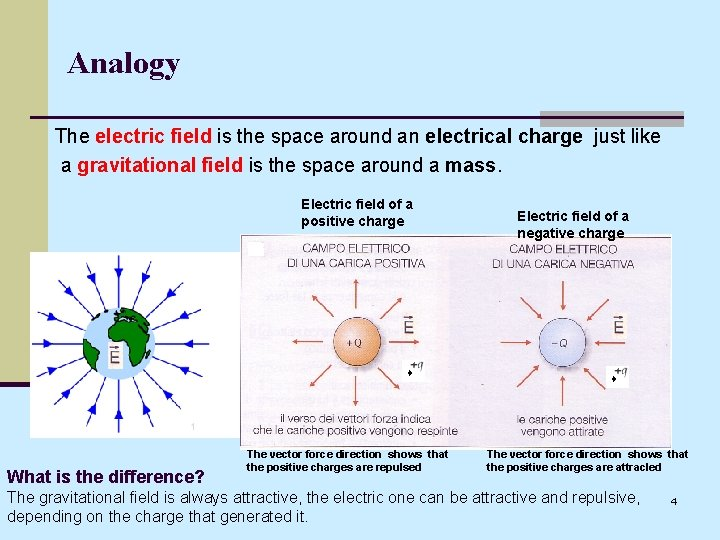Analogy The electric field is the space around an electrical charge just like a gravitational field is the space around a mass. Electric field of a positive charge What is the difference? The vector force direction shows that the positive charges are repulsed Electric field of a negative charge The vector force direction shows that the positive charges are attracled The gravitational field is always attractive, the electric one can be attractive and repulsive, depending on the charge that generated it. 4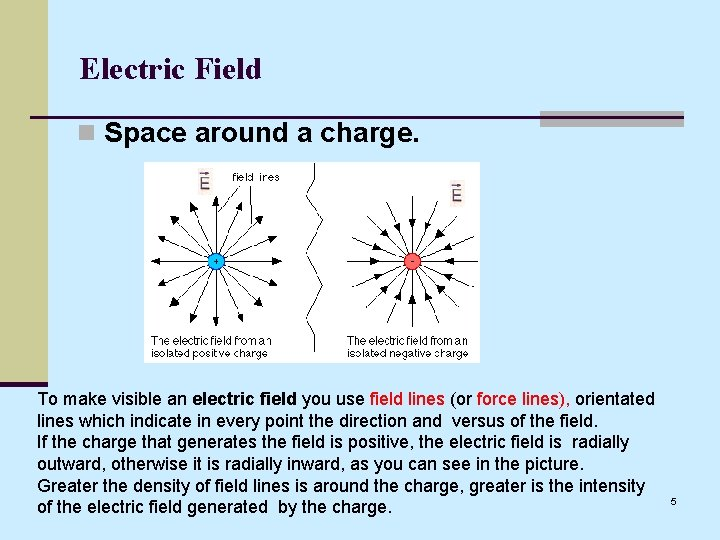Electric Field n Space around a charge. To make visible an electric field you use field lines (or force lines), orientated lines which indicate in every point the direction and versus of the field. If the charge that generates the field is positive, the electric field is radially outward, otherwise it is radially inward, as you can see in the picture. Greater the density of field lines is around the charge, greater is the intensity of the electric field generated by the charge. 5Electric Field Lines The electric field can be represented by field lines (or lines of force (force lines) ). By convention, these lines start on a positive charge and end on a negative charge. The charge on the right is twice the magnitude of the charge on the left (and opposite in sign), so there are twice as many field lines, and they point towards the charge rather than away from it. The number of field lines starting (ending) on a positive (negative) charge is proportional to the magnitude of the charge. Besides these lines, at each point, are tangent to the direction of the electric field. 6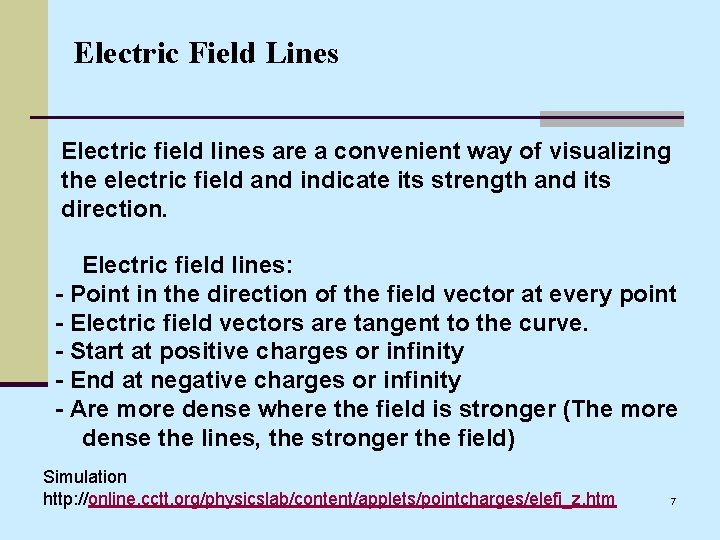Electric Field Lines Electric field lines are a convenient way of visualizing the electric field and indicate its strength and its direction. Electric field lines: - Point in the direction of the field vector at every point - Electric field vectors are tangent to the curve. - Start at positive charges or infinity - End at negative charges or infinity - Are more dense where the field is stronger (The more dense the lines, the stronger the field) Simulation http: //online. cctt. org/physicslab/content/applets/pointcharges/elefi_z. htm 7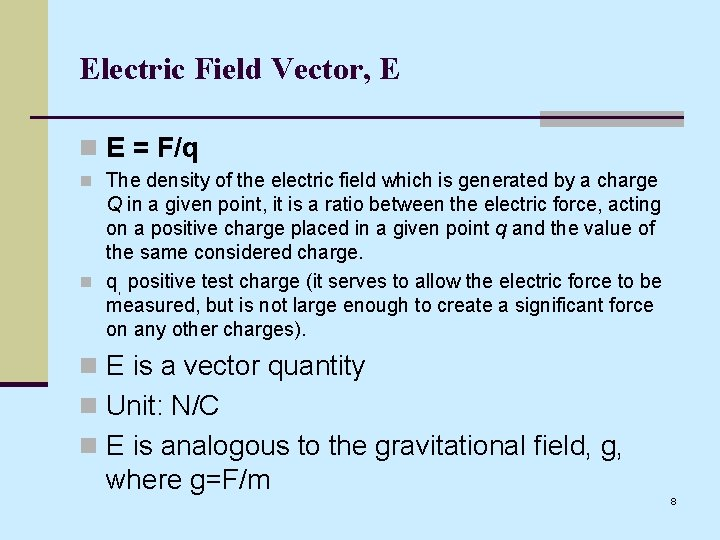Electric Field Vector, E n E = F/q n The density of the electric field which is generated by a charge Q in a given point, it is a ratio between the electric force, acting on a positive charge placed in a given point q and the value of the same considered charge. n q, positive test charge (it serves to allow the electric force to be measured, but is not large enough to create a significant force on any other charges). n E is a vector quantity n Unit: N/C n E is analogous to the gravitational field, g, where g=F/m 8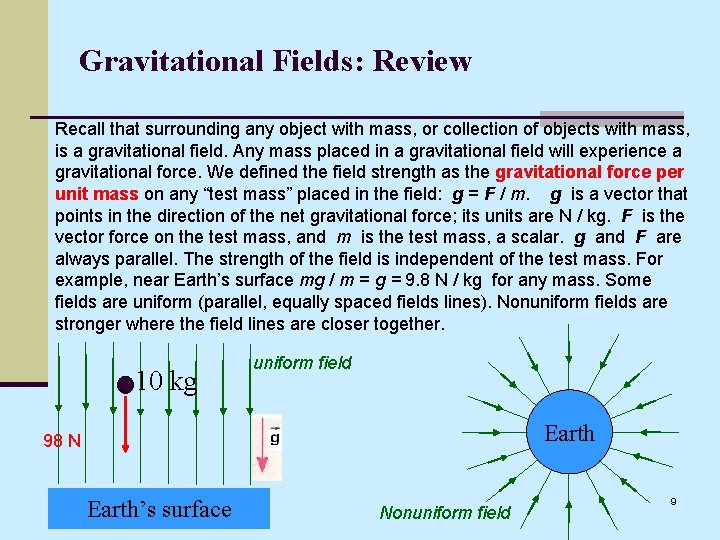Gravitational Fields: Review Recall that surrounding any object with mass, or collection of objects with mass, is a gravitational field. Any mass placed in a gravitational field will experience a gravitational force. We defined the field strength as the gravitational force per unit mass on any “test mass” placed in the field: g = F / m. g is a vector that points in the direction of the net gravitational force; its units are N / kg. F is the vector force on the test mass, and m is the test mass, a scalar. g and F are always parallel. The strength of the field is independent of the test mass. For example, near Earth’s surface mg / m = g = 9. 8 N / kg for any mass. Some fields are uniform (parallel, equally spaced fields lines). Nonuniform fields are stronger where the field lines are closer together. 10 kg uniform field Earth 98 N Earth’s surface Nonuniform field 9Electric Fields: Surrounding any object with charge, or collection of objects with charge, is a electric field. Any charge placed in an electric field will experience a electrical force. We defined the field strength as the electric force per unit charge on any “test charge” placed in the field: E = F / q. E is a vector that points, by definition, in the direction of the net electric force on a positive charge; its units are N / C. F is the vector force on the test charge, and q is the test charge, a scalar. E and F are only parallel if the test charge is positive. Some fields are uniform (parallel, equally spaced fields lines) such as the field on the left formed by a sheet of negative charge. Nonuniform fields are stronger where the field lines are closer together, such as the field on the right produced by a sphere of negative charge. uniform field +q - F ------- Nonuniform field 10Overview of Fields Charge, like mass, is an intrinsic property of an object. Charges produce electric fields that affect other charges; masses produce gravitational fields that affect other masses. Gravitational fields lines always point toward an isolated mass. Unlike mass, though, charges can be positive or negative. Electric field lines emanate from positive charges and penetrate into negative charge. We refer to the charge producing a field as a field charge. A group of field charges can produce very nonuniform fields. To determine the strength of the field at a particular point, we place a small, positive test charge in the field. We then measure the electric force on it and divide by the test charge: For an isolated positive field charge, the field lines point away from the field charge (since the force on a positive charge would be away from the field charge). The opposite is true for an isolated negative field charge. No matter how complex the field, the electric force on a test charge is always tangent to the field line at that point. The coming slides will reiterate these ideas and provide examples. 11Electric & Gravitational - Fields Compared Field strength Force Intrinsic Property SI units Gravity: g = P / m N / kg Electric Force: E = F / q N/C Field strength is given by per unit mass or force per unit charge, depending on the type of field. Field strength means the magnitude of a field vector. Ex #1: If a +10 C charge is placed in an electric field and experiences a 50 N force, the field strength at the location of the charge is 5 N/C. The electric field vector is given by: E = 5 N/C, where the direction of this vector is parallel to the force vector (and the field lines). Ex #2: If a -10 C charge experiences a 50 N force, E = 5 N/C in a direction opposite the force vector (opposite the direction of the field lines). 12Electric & Gravitational Fields - Compared Field Typical Vector Typical Force Potential Energy Electric Earth Gravitational Element on which the electric field acts 1 - Only if the field is uniform 2 - Only near the Earth surface where the field is almost uniform 13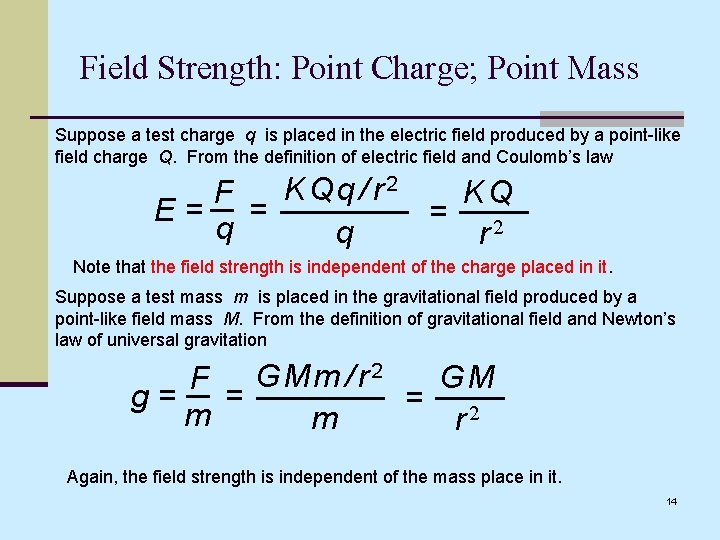Field Strength: Point Charge; Point Mass Suppose a test charge q is placed in the electric field produced by a point-like field charge Q. From the definition of electric field and Coulomb’s law K Qq / r 2 KQ F E= = = q q r 2 Note that the field strength is independent of the charge placed in it. Suppose a test mass m is placed in the gravitational field produced by a point-like field mass M. From the definition of gravitational field and Newton’s law of universal gravitation GM F G Mm / r 2 g= = = 2 m m r Again, the field strength is independent of the mass place in it. 14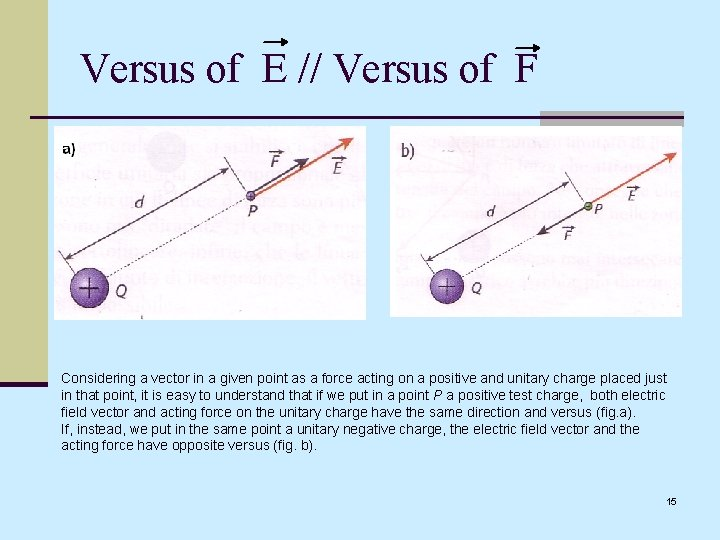Versus of E // Versus of F Considering a vector in a given point as a force acting on a positive and unitary charge placed just in that point, it is easy to understand that if we put in a point P a positive test charge, both electric field vector and acting force on the unitary charge have the same direction and versus (fig. a). If, instead, we put in the same point a unitary negative charge, the electric field vector and the acting force have opposite versus (fig. b). 15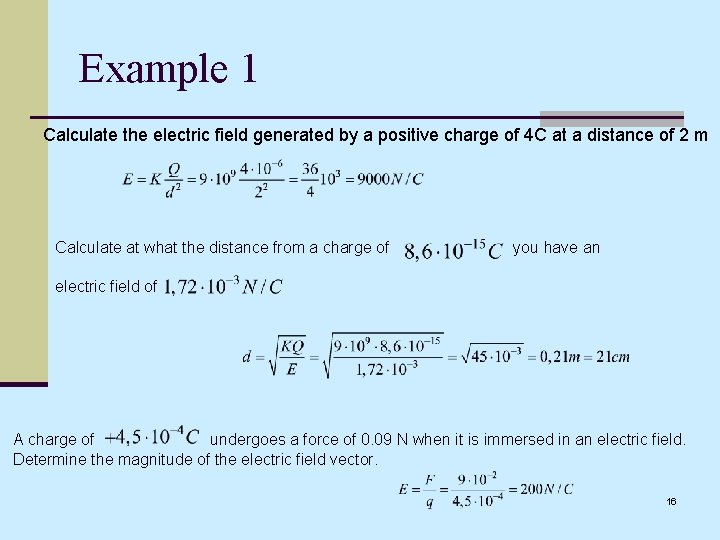Example 1 Calculate the electric field generated by a positive charge of 4 C at a distance of 2 m Calculate at what the distance from a charge of you have an electric field of A charge of undergoes a force of 0. 09 N when it is immersed in an electric field. Determine the magnitude of the electric field vector. 16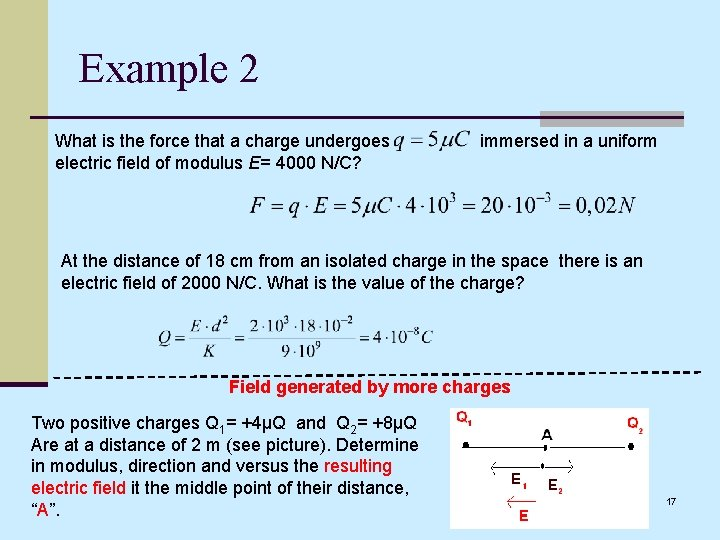Example 2 What is the force that a charge undergoes electric field of modulus E= 4000 N/C? immersed in a uniform At the distance of 18 cm from an isolated charge in the space there is an electric field of 2000 N/C. What is the value of the charge? Field generated by more charges Two positive charges Q 1= +4μQ and Q 2= +8μQ Are at a distance of 2 m (see picture). Determine in modulus, direction and versus the resulting electric field it the middle point of their distance, “A”. 17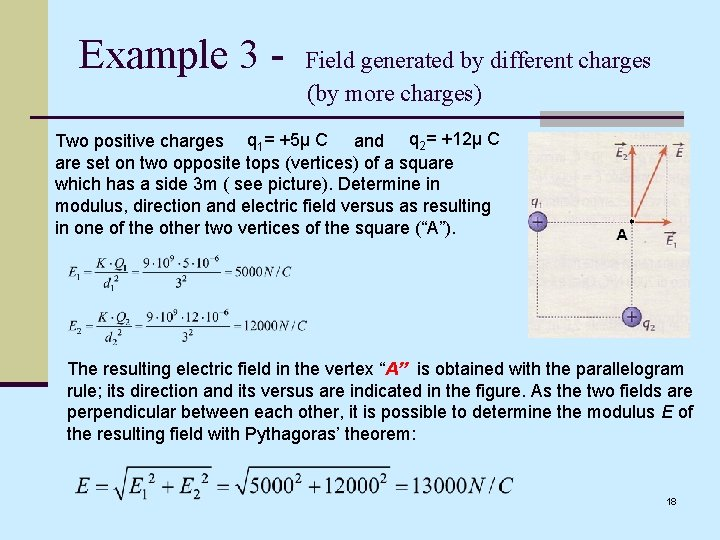Example 3 - Field generated by different charges (by more charges) Two positive charges q 1= +5μ C and q 2= +12μ C are set on two opposite tops (vertices) of a square which has a side 3 m ( see picture). Determine in modulus, direction and electric field versus as resulting in one of the other two vertices of the square (“A”). The resulting electric field in the vertex “A” is obtained with the parallelogram rule; its direction and its versus are indicated in the figure. As the two fields are perpendicular between each other, it is possible to determine the modulus E of the resulting field with Pythagoras’ theorem: 18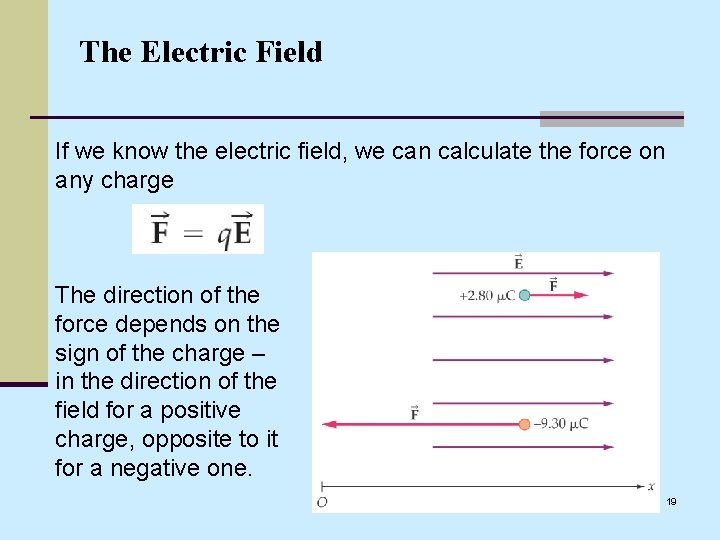The Electric Field If we know the electric field, we can calculate the force on any charge The direction of the force depends on the sign of the charge – in the direction of the field for a positive charge, opposite to it for a negative one. 19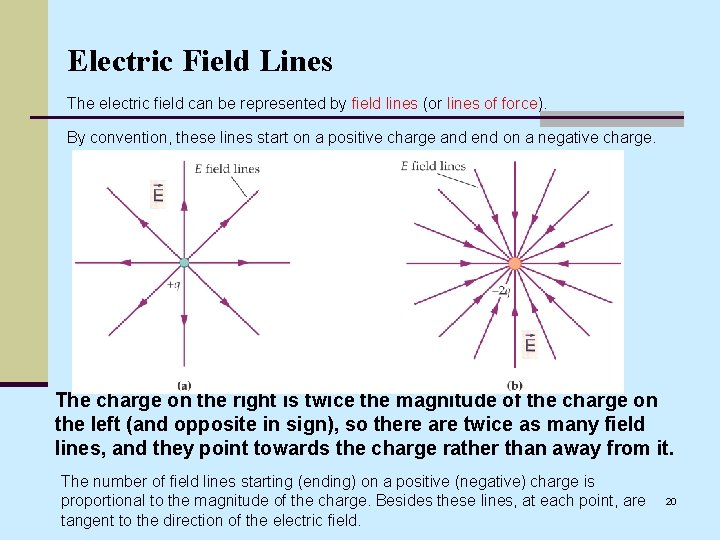Electric Field Lines The electric field can be represented by field lines (or lines of force). By convention, these lines start on a positive charge and end on a negative charge. The charge on the right is twice the magnitude of the charge on the left (and opposite in sign), so there are twice as many field lines, and they point towards the charge rather than away from it. The number of field lines starting (ending) on a positive (negative) charge is proportional to the magnitude of the charge. Besides these lines, at each point, are tangent to the direction of the electric field. 20Electric Field Lines Field generated by two point charges of opposite and equal in sign. Electric dipole: two equal charges, opposite in sign. - The direction of the electric field at any point is tangential to the field line through that point. - The magnitude of the electric field is given by the density of the fields lines. 21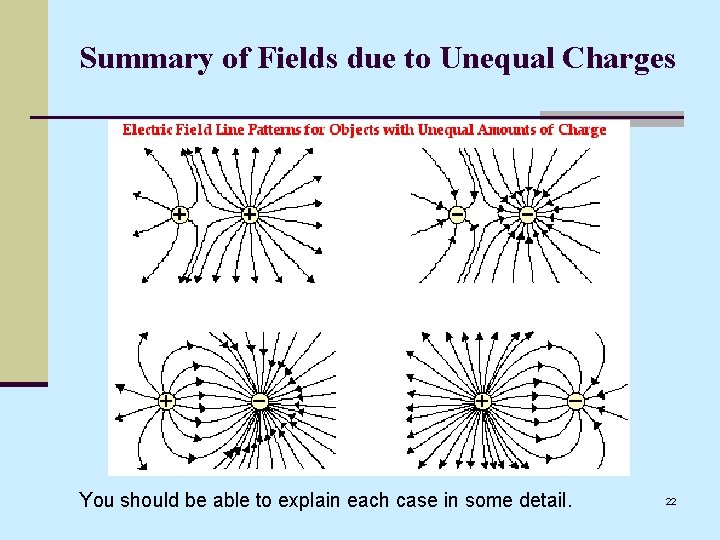Summary of Fields due to Unequal Charges You should be able to explain each case in some detail. 22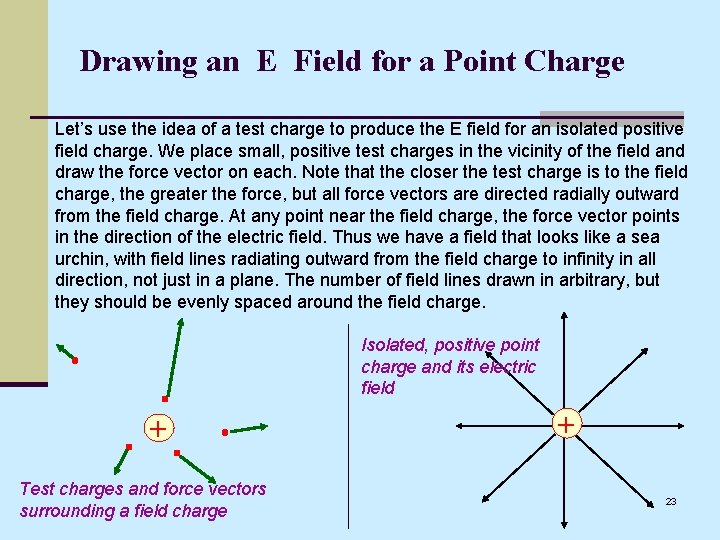Drawing an E Field for a Point Charge Let’s use the idea of a test charge to produce the E field for an isolated positive field charge. We place small, positive test charges in the vicinity of the field and draw the force vector on each. Note that the closer the test charge is to the field charge, the greater the force, but all force vectors are directed radially outward from the field charge. At any point near the field charge, the force vector points in the direction of the electric field. Thus we have a field that looks like a sea urchin, with field lines radiating outward from the field charge to infinity in all direction, not just in a plane. The number of field lines drawn in arbitrary, but they should be evenly spaced around the field charge. Isolated, positive point charge and its electric field + Test charges and force vectors surrounding a field charge + 23Point Charges of Different Magnitudes Let’s compare the fields on two separate isolated point charges, one with a charge of +1 unit, the other with a charge of +2 units. It doesn’t matter how many field lines we draw emanating from the +1 charge so long as we draw twice as many line coming from the +2 charge. This means, at a given distance, the strength of the E field for the +2 charge is twice that for the +1 charge. +1 +2 24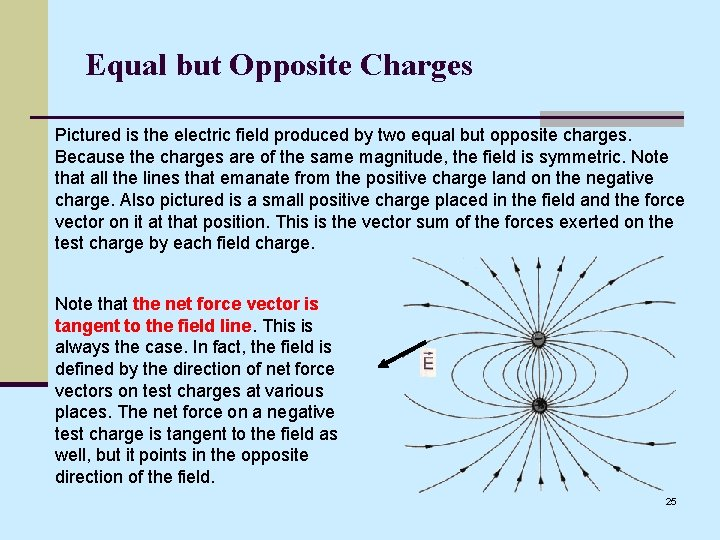Equal but Opposite Charges Pictured is the electric field produced by two equal but opposite charges. Because the charges are of the same magnitude, the field is symmetric. Note that all the lines that emanate from the positive charge land on the negative charge. Also pictured is a small positive charge placed in the field and the force vector on it at that position. This is the vector sum of the forces exerted on the test charge by each field charge. Note that the net force vector is tangent to the field line. This is always the case. In fact, the field is defined by the direction of net force vectors on test charges at various places. The net force on a negative test charge is tangent to the field as well, but it points in the opposite direction of the field. 25Equal but Opposite Charges (cont. ) D C - + A B Here is another view of the field. Since the net force on a charge can only be in one direction, field lines never intersect. Draw the electric force on a positive charge at A, the electric field vector and B, and the electric force on a negative charge at C. The net force on a + charge at D charge is directly to the left. Show why this is the case by 26 drawing force vectors from each field charge and then summing these vectors.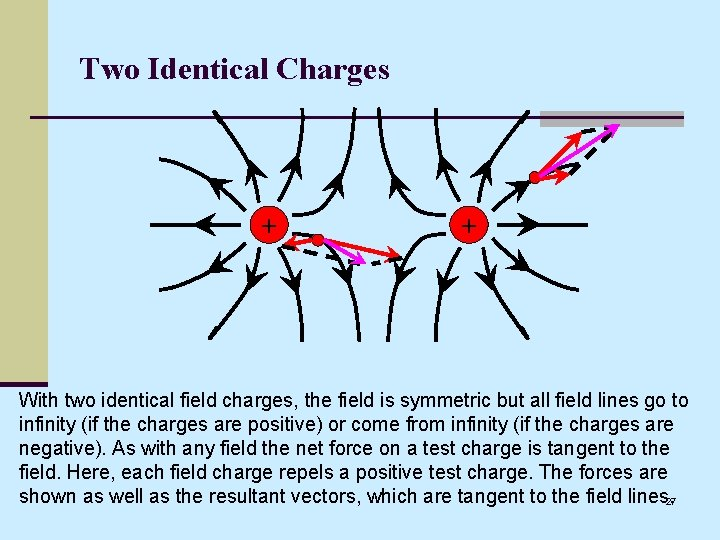Two Identical Charges + + With two identical field charges, the field is symmetric but all field lines go to infinity (if the charges are positive) or come from infinity (if the charges are negative). As with any field the net force on a test charge is tangent to the field. Here, each field charge repels a positive test charge. The forces are shown as well as the resultant vectors, which are tangent to the field lines. 27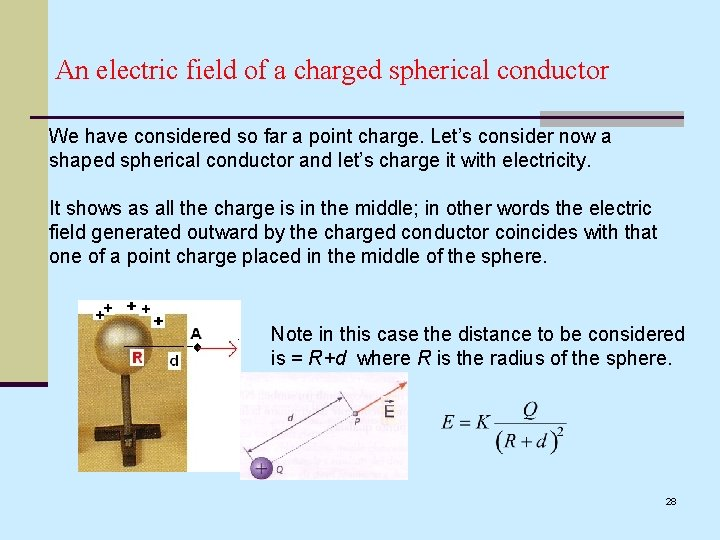An electric field of a charged spherical conductor We have considered so far a point charge. Let’s consider now a shaped spherical conductor and let’s charge it with electricity. It shows as all the charge is in the middle; in other words the electric field generated outward by the charged conductor coincides with that one of a point charge placed in the middle of the sphere. Note in this case the distance to be considered is = R+d where R is the radius of the sphere. 28In a spherical conductor the charge arranges in a uniform way on the outward surface and the electric field on its inward is: E = 0. E=0. Note that the lines of the electric field, generated outward by a charge Q which is uniform distributed on a spherical conductor, are parallel between them and are perpendicular to the surface of the conductor. Two simple experiments make clearer the idea. 29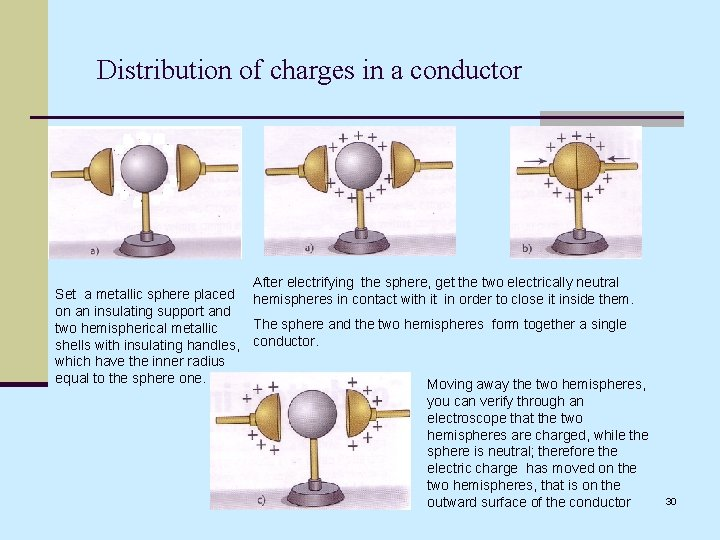Distribution of charges in a conductor After electrifying the sphere, get the two electrically neutral Set a metallic sphere placed hemispheres in contact with it in order to close it inside them. on an insulating support and The sphere and the two hemispheres form together a single two hemispherical metallic shells with insulating handles, conductor. which have the inner radius equal to the sphere one. Moving away the two hemispheres, you can verify through an electroscope that the two hemispheres are charged, while the sphere is neutral; therefore the electric charge has moved on the two hemispheres, that is on the outward surface of the conductor 30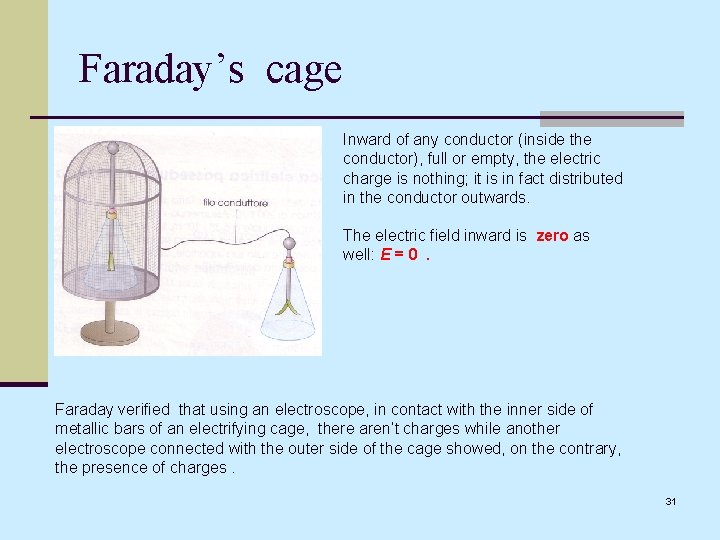Faraday’s cage Inward of any conductor (inside the conductor), full or empty, the electric charge is nothing; it is in fact distributed in the conductor outwards. The electric field inward is zero as well: E = 0. Faraday verified that using an electroscope, in contact with the inner side of metallic bars of an electrifying cage, there aren’t charges while another electroscope connected with the outer side of the cage showed, on the contrary, the presence of charges. 31Charged no- spherical conductor In a no- spherical conductor the charges gather on the points where the surface is more curved, here the intensity of the field is greater and the lines thicken An extreme condition is that of the points, where the electric field is very intense. The lightning conductor, with its point-shaped, attracts the lightning, but thanks to its conductor cable, comes into contact with the ground, discharging on it the huge electric energy the lightning gives it up so protecting the house. During a storm, therefore, it is safer to stay in the car, which acts as a screen; otherwise , if you are outdoor never get a shelter under a tree or stand in an open field. 32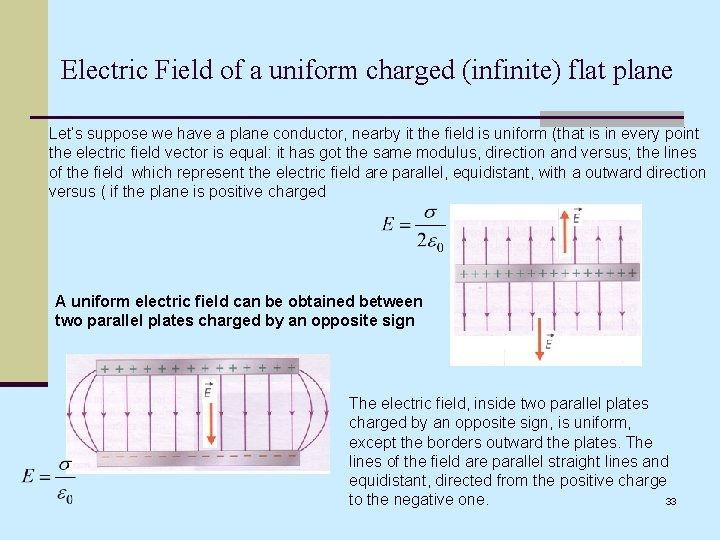Electric Field of a uniform charged (infinite) flat plane Let’s suppose we have a plane conductor, nearby it the field is uniform (that is in every point the electric field vector is equal: it has got the same modulus, direction and versus; the lines of the field which represent the electric field are parallel, equidistant, with a outward direction versus ( if the plane is positive charged A uniform electric field can be obtained between two parallel plates charged by an opposite sign The electric field, inside two parallel plates charged by an opposite sign, is uniform, except the borders outward the plates. The lines of the field are parallel straight lines and equidistant, directed from the positive charge to the negative one. 33Field generated by two charged parallel flat plates A positive charge q and a mass m, with a starting speed v perpendicular to the field, describes a bending parabola towards the versus of the field. The acceleration a depends on m. A negative charge q and a mass m, with a starting speed v perpendicular to the field, describes a bending parabola towards the opposite versus of the field. If the mass or the charge changes, the deflection of the charged body also changes, compared to its starting motion direction. 34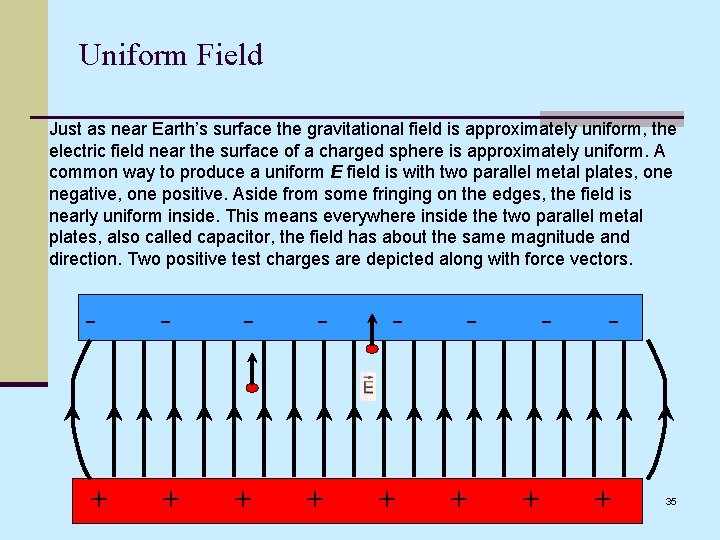Uniform Field Just as near Earth’s surface the gravitational field is approximately uniform, the electric field near the surface of a charged sphere is approximately uniform. A common way to produce a uniform E field is with two parallel metal plates, one negative, one positive. Aside from some fringing on the edges, the field is nearly uniform inside. This means everywhere inside the two parallel metal plates, also called capacitor, the field has about the same magnitude and direction. Two positive test charges are depicted along with force vectors. - - - + + + - + 35Opposite Signs, Unequal Charges The positive charge has a greater magnitude than the negative charge. Explain why the field is as shown. (Answer on next slide. ) + - 36Opposite Signs, Unequal Charges (cont. ) + - More field lines come from the positive charge than land on the negative. Those that don’t land on the negative charge go to infinity. As always, net force on a test charge is the vector sum of the two forces and it’s tangent to the field. Since the positive charge has greater magnitude, it dominates the negative charge, forcing the “turning points” of the point to be closer to the negative charge. If you were to “zoom out” (observe the field from a distance) it would look like that of an isolated, positive point 37 with a charge equal to the net charge of the system.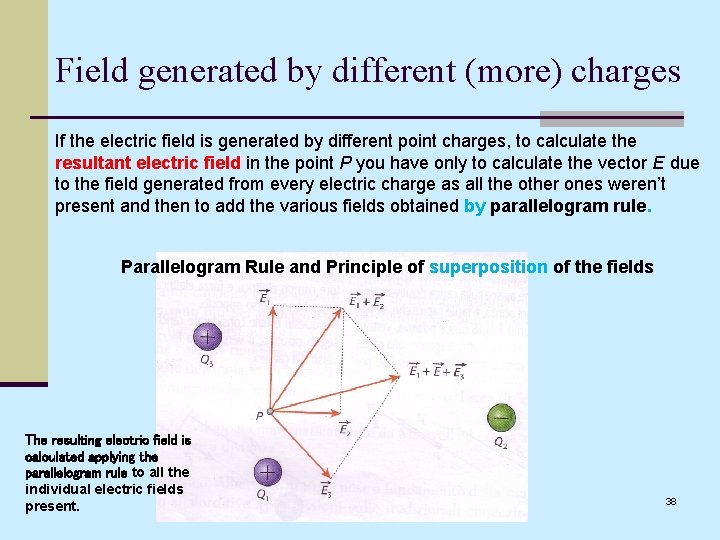Field generated by different (more) charges If the electric field is generated by different point charges, to calculate the resultant electric field in the point P you have only to calculate the vector E due to the field generated from every electric charge as all the other ones weren’t present and then to add the various fields obtained by parallelogram rule. Parallelogram Rule and Principle of superposition of the fields The resulting electric field is calculated applying the parallelogram rule to all the individual electric fields present. 38Flusso del campo Elettrico Si definisce flusso del campo elettrico E, attraverso la superficie S, il numero di linee del campo che attraversano tale superficie. S è la superficie disposta perpendicolarmente ad E, altrimenti : Se la superfici è disposta parallelamente ad E, il flusso vale zero. Il flusso si misura in : Volt x metro 39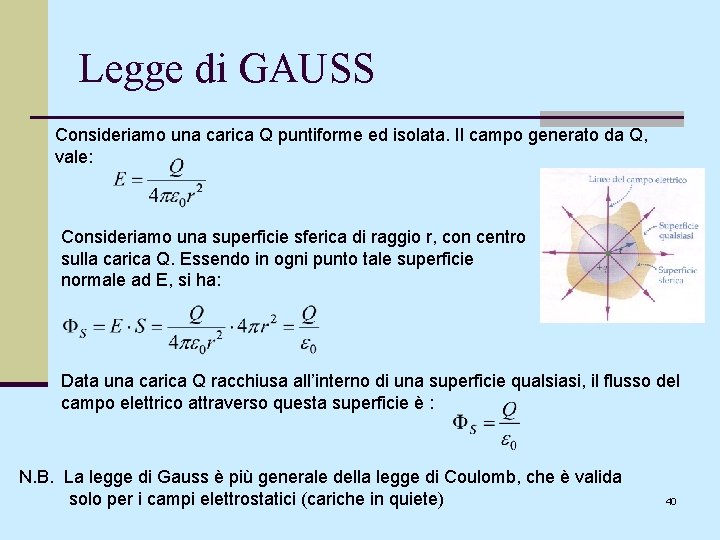Legge di GAUSS Consideriamo una carica Q puntiforme ed isolata. Il campo generato da Q, vale: Consideriamo una superficie sferica di raggio r, con centro sulla carica Q. Essendo in ogni punto tale superficie normale ad E, si ha: Data una carica Q racchiusa all’interno di una superficie qualsiasi, il flusso del campo elettrico attraverso questa superficie è : N. B. La legge di Gauss è più generale della legge di Coulomb, che è valida solo per i campi elettrostatici (cariche in quiete) 40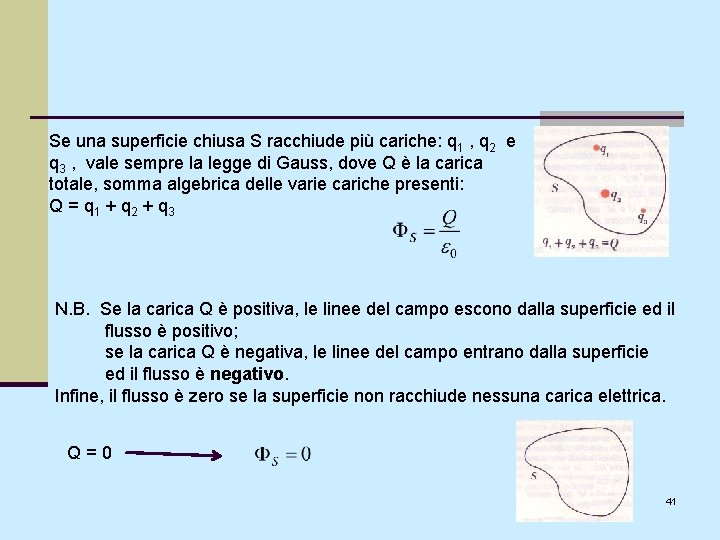Se una superficie chiusa S racchiude più cariche: q 1 , q 2 e q 3 , vale sempre la legge di Gauss, dove Q è la carica totale, somma algebrica delle varie cariche presenti: Q = q 1 + q 2 + q 3 N. B. Se la carica Q è positiva, le linee del campo escono dalla superficie ed il flusso è positivo; se la carica Q è negativa, le linee del campo entrano dalla superficie ed il flusso è negativo. Infine, il flusso è zero se la superficie non racchiude nessuna carica elettrica. Q=0 41Conseguenze della legge di Gauss 1) Un conduttore sferico isolato, con carica Q, genera lo stesso campo elettrico che sarebbe generato se la carica Q fosse concentrata nel centro della sfera; Consideriamo la legge di Gauss applicata alla superficie sferica S del conduttore sferico carico: La formula trovata è quella del campo elettrico creato da una carica ad una distanza r dalla carica stessa. 42Conseguenze della legge di Gauss 2) La carica elettrica di un conduttore in equilibrio elettrico (o elettrostatico) è localizzata sulla superficie; Sia C un conduttore di superficie S con carica Q. Internamente è (per equilibrio elettrico): E = 0 ; quindi il flusso attraverso una qualsiasi superficie interna è zero. Consideriamo una superficie S’ chiusa interna ad S; si ha che: Ciò vuol dire, per la legge di Gauss, che Q = 0, cioè non esistono all’interno cariche non equilibrate. Dato che S’ si può avvicinare ad S quanto si vuole, ne viene che le cariche in eccesso debbono trovarsi sulla superficie del conduttore. 43Conseguenze della legge di Gauss 3) Il campo elettrico tra due armature piane: Consideriamo come superficie gaussiana un cilindro che attraversa una delle armature, come da figura. - il flusso attraverso la superficie laterale è zero, perché la superficie è parallela al campo elettrico; -Il flusso attraverso la base di sinistra è nullo, perché il campo elettrico all’interno delle armature è zero; - l’unico contributo è quello della base di destra del cilindro, posta tra le armature; il flusso vale: In base alla definizione, sappiamo che: per cui, si ha: cioè: 44Conseguenze della legge di Gauss 4) La capacità di un condensatore piano è: Consideriamo come superficie gaussiana un cilindro che attraversa una delle armature come da figura. - il flusso attraverso la superficie laterale è zero, perché la superficie è parallela al campo elettrico; -Il flusso attraverso la base di sinistra è nullo, perché il campo elettrico all’interno delle armature è zero; - l’unico contributo è quello della base di destra del cilindro, posta tra le armature; il flusso vale: cioè: Noi sappiamo che: cioè: 45Conseguenze della legge di Gauss 5) La legge di Coulomb Una carica q 2 crea nel punto P il campo elettrico E. La forza che agisce su di una carica q 1 pota nel punto P vale: (1) Consideriamo la legge di Gauss, prendendo in esame come superficie S, chiusa, la sfera di raggio r con centro nella carica q 2, abbiamo: Sostituiamo tale valore nella relazione (1) , ed abbiamo: che è la legge di Coulomb. 46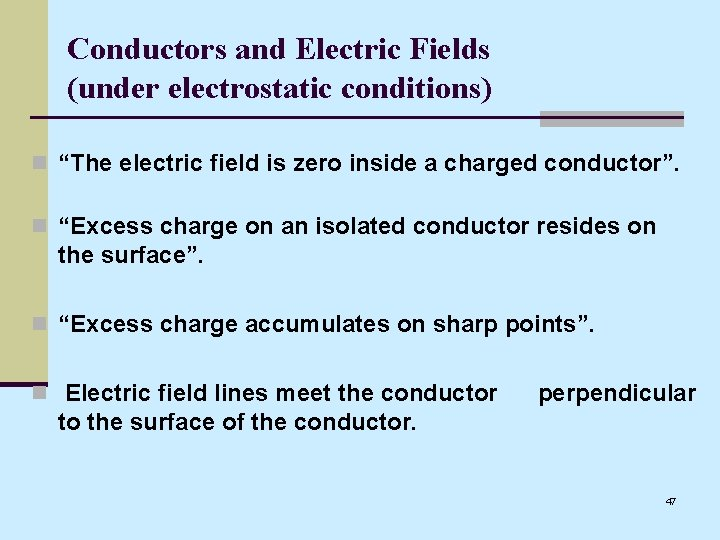Conductors and Electric Fields (under electrostatic conditions) n “The electric field is zero inside a charged conductor”. n “Excess charge on an isolated conductor resides on the surface”. n “Excess charge accumulates on sharp points”. n Electric field lines meet the conductor perpendicular to the surface of the conductor. 47Shielding Where are you safe during a thunderstorm? A) In a car or B) Outdoors Why can you not get radio reception in a tunnel or in a steel bridge? 48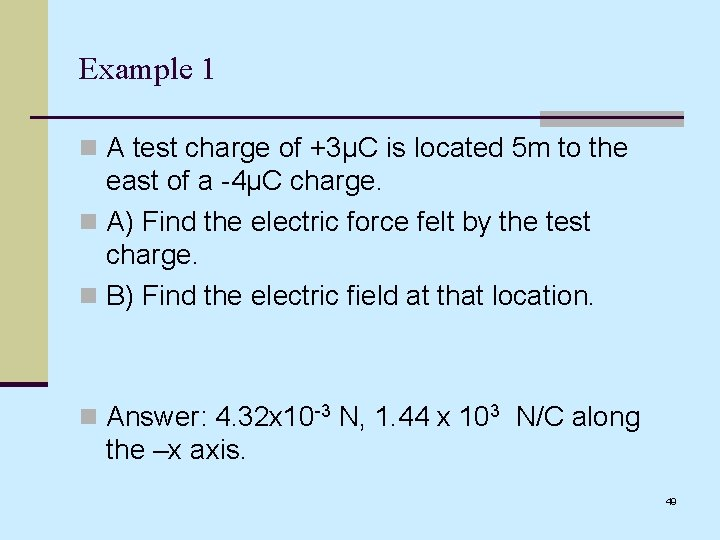Example 1 n A test charge of +3µC is located 5 m to the east of a -4µC charge. n A) Find the electric force felt by the test charge. n B) Find the electric field at that location. n Answer: 4. 32 x 10 -3 N, 1. 44 x 103 N/C along the –x axis. 49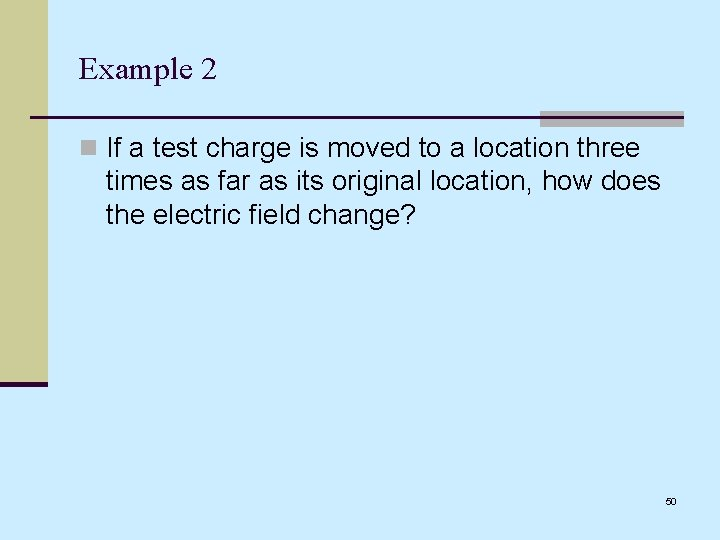Example 2 n If a test charge is moved to a location three times as far as its original location, how does the electric field change? 50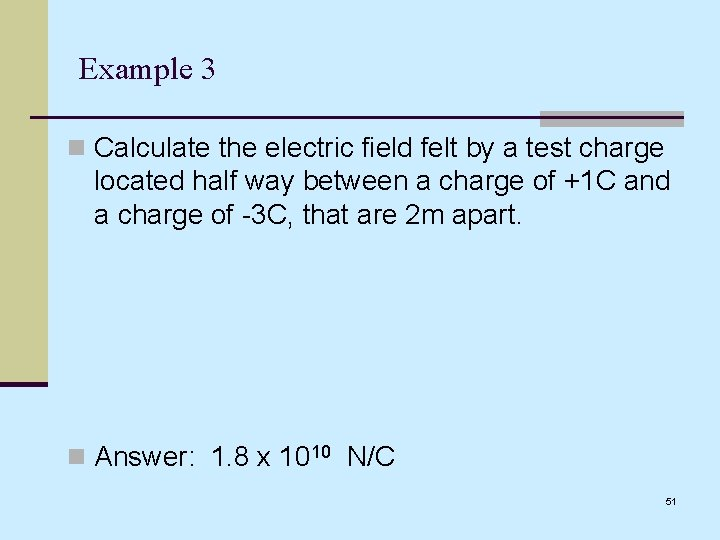Example 3 n Calculate the electric field felt by a test charge located half way between a charge of +1 C and a charge of -3 C, that are 2 m apart. n Answer: 1. 8 x 1010 N/C 51When electric charges are at rest, the electric field within a conductor is zero. 52The electric field is always perpendicular to the surface of a conductor – if it weren’t, the charges would move along the surface. 53Excess Charge on a Conductor Any excess charge placed on a conductor will immediately distribute itself over the surface of the conductor. No excess charge will remain inside. On a spherical conductor the excess charge will be distributed evenly. If electrons are added, they themselves will spread out. If electrons are removed, electrons in the conductor will replace them, leaving all excess positive charge on the surface. Excess charge placed on an insulator pretty much stays put. + + _ _ + _ + __ _ Now lets add some extra charges. The new charges repel themselves and reside only on the surface. 54Excess Charge on a Pointy Conductor Excess charge, which always resides on the surface of a conductor, will collect in high concentrations at points. In general, the smaller the radius of curvature, R, the greater the charge density (charge per unit area). The reason for this is that when R is large, neighboring charges push a charge nearly tangent to the surface (left pic). But where R is small (as near a point), neighboring charges are mostly pushing a charge outward, away from the surface instead of away from each other (right pic). This allows the charges be reside closer together. _ _ _ _ _ vector forces due to neighboring charges _ _ _ _ _ small R, high charge density _ _ __ _ _ _ large R, low charge density _ _ _ 55Electric Fields In & Around Charged Conductors E is always zero inside any conductor, even a charged one. If this were not the case, mobile valence electrons inside the conductor would be accelerated by the E field, leaving them in a state of perpetual motion. Outside a charged conductor E is greater where the charge density is greater. Near points, E can be extremely high. Surrounding a sphere the field is radially symmetric, just the field due to a point charge. _ _ _ _ _ E=0 inside _ _ _ _ _ small R, strong E _ _ _ large R, weak E _ __ _ _ _ E=0 inside _ _ _ 56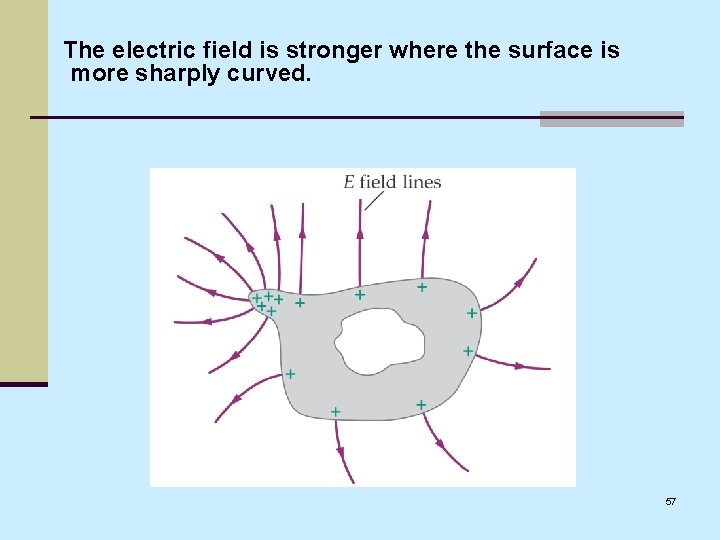The electric field is stronger where the surface is more sharply curved. 57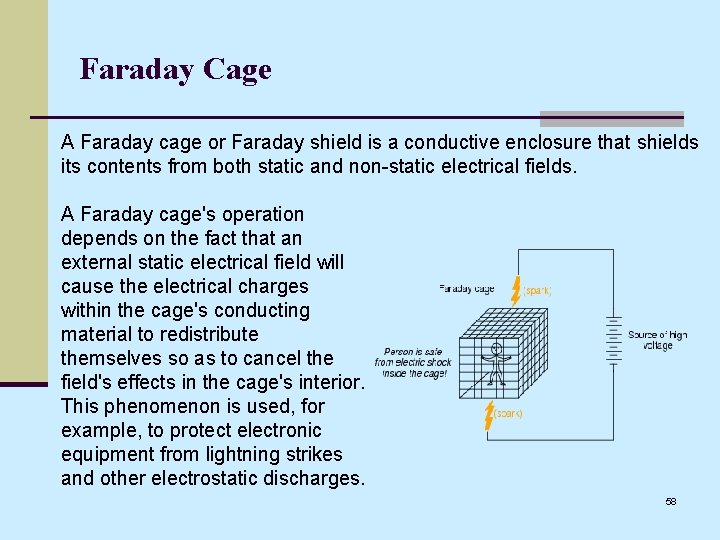Faraday Cage A Faraday cage or Faraday shield is a conductive enclosure that shields its contents from both static and non-static electrical fields. A Faraday cage's operation depends on the fact that an external static electrical field will cause the electrical charges within the cage's conducting material to redistribute themselves so as to cancel the field's effects in the cage's interior. This phenomenon is used, for example, to protect electronic equipment from lightning strikes and other electrostatic discharges. 58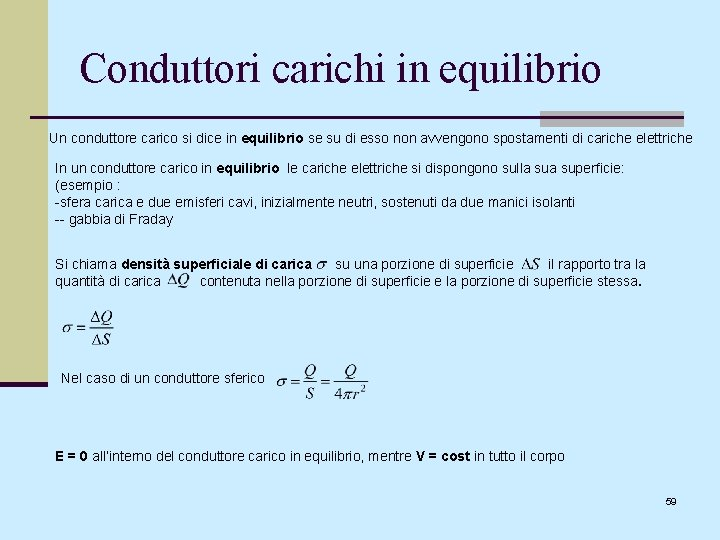Conduttori carichi in equilibrio Un conduttore carico si dice in equilibrio se su di esso non avvengono spostamenti di cariche elettriche In un conduttore carico in equilibrio le cariche elettriche si dispongono sulla superficie: (esempio : -sfera carica e due emisferi cavi, inizialmente neutri, sostenuti da due manici isolanti -- gabbia di Fraday Si chiama densità superficiale di carica su una porzione di superficie il rapporto tra la quantità di carica contenuta nella porzione di superficie e la porzione di superficie stessa. Nel caso di un conduttore sferico E = 0 all’interno del conduttore carico in equilibrio, mentre V = cost in tutto il corpo 59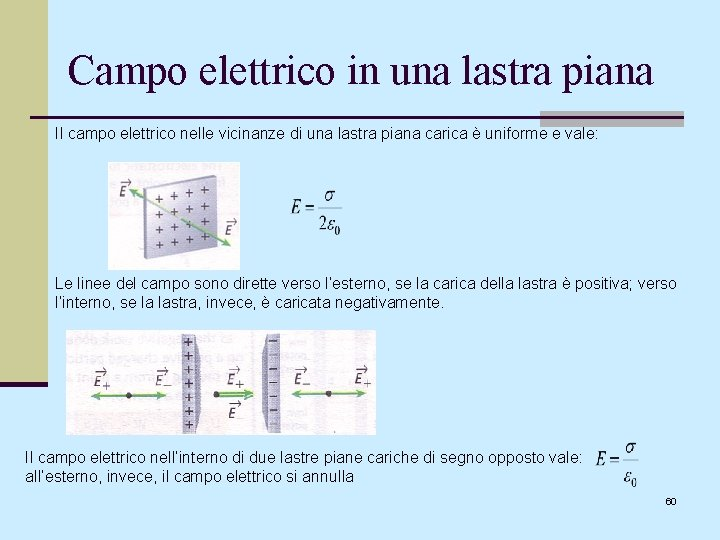Campo elettrico in una lastra piana Il campo elettrico nelle vicinanze di una lastra piana carica è uniforme e vale: Le linee del campo sono dirette verso l’esterno, se la carica della lastra è positiva; verso l’interno, se la lastra, invece, è caricata negativamente. Il campo elettrico nell’interno di due lastre piane cariche di segno opposto vale: all’esterno, invece, il campo elettrico si annulla 60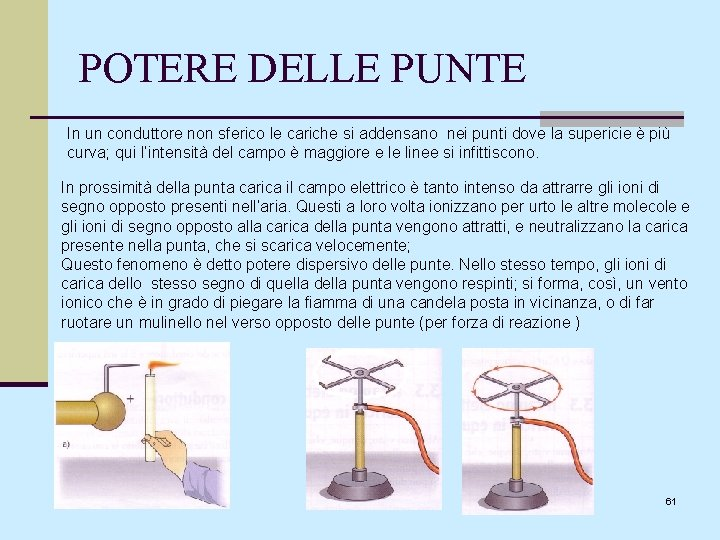POTERE DELLE PUNTE In un conduttore non sferico le cariche si addensano nei punti dove la supericie è più curva; qui l’intensità del campo è maggiore e le linee si infittiscono. In prossimità della punta carica il campo elettrico è tanto intenso da attrarre gli ioni di segno opposto presenti nell’aria. Questi a loro volta ionizzano per urto le altre molecole e gli ioni di segno opposto alla carica della punta vengono attratti, e neutralizzano la carica presente nella punta, che si scarica velocemente; Questo fenomeno è detto potere dispersivo delle punte. Nello stesso tempo, gli ioni di carica dello stesso segno di quella della punta vengono respinti; si forma, così, un vento ionico che è in grado di piegare la fiamma di una candela posta in vicinanza, o di far ruotare un mulinello nel verso opposto delle punte (per forza di reazione ) 61